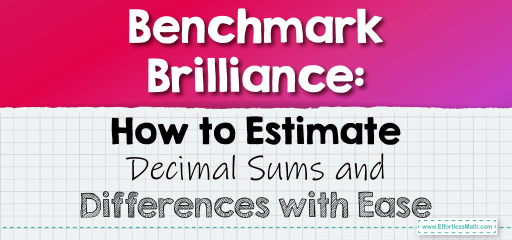# Benchmark Brilliance: How to Estimate Decimal Sums and Differences with Ease

Benchmarks are reference points that help us make quick estimates. Common benchmarks for decimals include $$0$$, $$0.5$$, and $$1$$. By rounding decimals to these benchmarks, we can swiftly estimate their sums and differences. Let's delve into how to use benchmarks for estimating decimal operations.## Estimating Sums and Differences of Decimals Using Benchmarks

### Example 1:

Estimate the sum of $$0.47$$ and $$0.68$$.

Estimation Process Using Benchmarks:

1. Round $$0.47$$ to $$0.5$$.

2. Round $$0.68$$ to $$0.5$$.

3. Estimate the sum: $$0.5 + 0.5 = 1$$.

The estimated sum is $$1$$.

The Absolute Best Book for 5th Grade Students

### Example 2:

Estimate the difference between $$0.72$$ and $$0.29$$.

Estimation Process Using Benchmarks:

1. Round $$0.72$$ to $$0.5$$.

2. Round $$0.29$$ to $$0.5$$.

3. Estimate the difference: $$0.5 – 0.5 = 0$$.

The estimated difference is $$0$$.

Using benchmarks to estimate sums and differences of decimals provides a quick and intuitive approach to understanding the approximate outcome of operations. This method is especially useful when you need a general idea rather than precise calculations. By rounding to the nearest benchmark and then performing the operation, you can get a ballpark figure swiftly. Practice regularly to master this invaluable skill.

### Practice Questions:

1. Estimate the sum of $$0.56$$ and $$0.44$$ using benchmarks.

2. What is the estimated difference between $$0.87$$ and $$0.34$$ using benchmarks?

3. Using benchmarks, estimate the sum of $$0.23$$ and $$0.79$$.

4. Estimate the difference between $$0.91$$ and $$0.46$$ using benchmarks.

5. Using benchmarks, what is the estimated sum of $$0.66$$ and $$0.31$$?

A Perfect Book for Grade 5 Math Word Problems!

1. $$1$$

2. $$0.5$$

3. $$1$$

4. $$0.5$$

5. $$1$$

The Best Math Books for Elementary Students

### What people say about "Benchmark Brilliance: How to Estimate Decimal Sums and Differences with Ease - Effortless Math: We Help Students Learn to LOVE Mathematics"?

No one replied yet.

X
51% OFF

Limited time only!

Save Over 51%

SAVE $15 It was$29.99 now it is \$14.99